# RRB ALP & Technician Mock Test (English) - 16

## 75 Questions MCQ Test RRB ALP & Technician Exam (Group C ) - Mock Tests | RRB ALP & Technician Mock Test (English) - 16

Description
Attempt RRB ALP & Technician Mock Test (English) - 16 | 75 questions in 60 minutes | Mock test for Railways preparation | Free important questions MCQ to study RRB ALP & Technician Exam (Group C ) - Mock Tests for Railways Exam | Download free PDF with solutions
QUESTION: 1

Solution:
QUESTION: 2

### The ratio between the present ages of P and Q is 5:7 respectively. If the difference between Q's present age and P's age after 6 years is 2, what is the total of P's and Q's present ages?

Solution:

Present ages of P and Q
= 5x years and 7x years
Then 7x - (5x + 6) = 2
2x = 8
∴ x = 4
∴ Required sum = 5x + 7x = 12x
= 12 x 4 = 48 years

QUESTION: 3

### Select the related word/figure from the given alternatives. Genuine : Authentic : : Mirage :?

Solution:
QUESTION: 4

What is the ratio of the areas of two circles whose radii are in the ratio 1:2 ?

Solution:

Let radius of one circle is x
So radius of other is 2x
Ratio of area = πx2/π(2x)2
= πx2/π4x2  = 1/4 = 1 : 4

QUESTION: 5
The general formula of an ester where R represents alkyl group is :
Solution:
QUESTION: 6
Consider the following organisms :
1. Agaricus
2. Aspergillus
3. Ulothrix
4. Ulva
Which of these are algae ?
Solution:
QUESTION: 7
How many days are there from 2nd January 1995 to 15th March, 1995 ?
Solution: 30 + 28 + 15 = 73 days
QUESTION: 8
The largest cell in the human body is
Solution:
QUESTION: 9
The correct formula of rust is
Solution:
QUESTION: 10
Choose the group of letters which is different from others.
Solution:
QUESTION: 11
Choose the word which is least like the other words in the group.
Solution:
QUESTION: 12
If BOMBAY is written as MYMYMY, how will TAMIL NADU be written in that code ?
Solution: The letters at the third and sixth places are repeated thrice to code BOMBAY as MYMYMY.
Similarly, the letters at the third, sixth and ninth places are repeated thrice to code TAMIL NADU as MNUMNUMNU.
QUESTION: 13
Pointing to a man, a lady says that his father is the third son of her grandmother. How is the lady related to that man ?
Solution: Pointing to a man a lady says that his father:

the third son of her grandmother:

Son of her grand mother means, that person is the lady's father or uncle.
Merge the two diagrams above. To the man the lady is pointing is son of one of these 3 sons.

If the lady and the man has children of two different people then they are cousins, otherwise they are siblings. So the lady is either cousin or sister to the man.
QUESTION: 14
If MOBILITY is coded as 46293927, then EXAMINATION is coded as :
Solution: Let A = 1,
B = 2,
C = 3,...., X = 24,
Y = 25,
Z = 26.
Then , M = 13 = 1 + 3 = 4;
O = 15 = 1 + 5 = 6;
L = 12 = 1 + 2 = 3;
T = 20 = 2 + 0 = 2;
Y = 25 = 2 + 5 = 7.
So, MOBILITY = 46293927
Similarly, EXAMINATION = 56149512965.
QUESTION: 15
The difference between the simple and the Compound Interest compounded every 6 months at the rate of 10 p.c.p.a at the end of 2 yrs is Rs 124.05. What is the sum?
Solution:
QUESTION: 16
Complete the analogous pair.
Leader : Follower : : Captain : ?
Solution:
QUESTION: 17
“Time’s Up” is a recently-launched Hollywood-project that will
Solution:
QUESTION: 18
The ‘Cancer train’ is synonymous with a majority of passengers belonging to the state of
Solution:
QUESTION: 19
Which among the following assets can be regarded as the most dependable platform for a second-strike, given the country’s “no first use” policy on nuclear weapons?
Solution:
QUESTION: 20
Which among the given has recently taken over majority stakes in a Mumbai-based start-up, CreditMate?
Solution:
QUESTION: 21
Who has been conferred with the prestigious National Design Award 2017 by the National Design Research Forum (NDRF)?
Solution:
QUESTION: 22
Each of the questions given below consists of a question and two statements numbered I and II given below it. You have to decide whether the data provided in the statements are sufficient to answer the questions.
How many students are there in a row?
Statements:
I. Manoj is the third from the right end.
II. Ashok is the fifth from the left end and thirteenth to the left of Manoj.
Solution:
Their are total 20 students
Hence, both the statements are necessary to answer the question.
QUESTION: 23
Mansur bin Muqrin who died in a helicopter crash recently was the Prince of which country?
Solution:
QUESTION: 24
The 150-megawatt Aurora plant, which is the world’s largest solar-thermal power plant, will be set up in which country?
Solution:
QUESTION: 25
A child is looking for his father. He went 90 metres in the East before turning to his right. He went 20 metres before turning to his right again to look for his father at his uncle's place 30 metres from this point. His father was not there. From here he went 100 metres to the North before meeting his father in a street. How far did the son meet his father from the starting point ?
Solution:
Required distance = AE
$=\sqrt{A{G}^{2}+E{G}^{2}}$
$=\sqrt{{\left(90-30\right)}^{2}+{\left(100-20\right)}^{2}}$
$=\sqrt{{\left(60\right)}^{2}+{\left(80\right)}^{2}}$
$=\sqrt{3600+6400}$ $=\sqrt{10000}$
$=100m$
QUESTION: 26
From a power-station ,the power is transmitted at a very high voltage because
Solution:
QUESTION: 27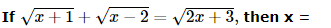Solution: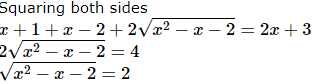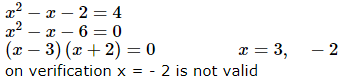QUESTION: 28

sin α = x/y , cos α = a/b , cot α =

Solution:
QUESTION: 29

Value of the following is cos230o + tan260o  − 3/4 sin245o  + cosec2300

Solution:
QUESTION: 30
Which physical quantity corresponds to the rate of change of momentum?
Solution: From Newtons second law Force is proportional to rate of change of momentum.
QUESTION: 31

54.327×357.2×0.0057 is the same as :

Solution:
QUESTION: 32
An angle which is greater than 180� but less than 360� is called a/an :
Solution:
QUESTION: 33
The locus of the mid-point of the equal chords of a given circle is :
Solution:
QUESTION: 34
Which of the following diseases is/are likely to spread in the entire community if a leaky septic tank contaminates the water supply? 1 Cholera 2 Typhoid 3 Tuberculosis 4 Leprosy
Solution:
QUESTION: 35
A satellite is revolving around the earth in the direction of the earth. A point on the equator of the earth is viewed by an observer from the satellite after every 8 hours. How much time does the satellite take to complete one revolution around the earth?
Solution:
QUESTION: 36
Consider the following statements regarding the living cell :
1. The Golgi apparatus links carbohydrates with proteins to form glycoproteins
2. In plants, the Golgi complex synthesizes pectin
3. The lysosomes store the hydrolysing enzymes
Which of these statements is/are correct ?
Solution:
QUESTION: 37
The least number which when divided by 25,40 and 60 leaves the remainder 7 in each case is
Solution:
QUESTION: 38
The Asia’s longest bi-directional Zojila Pass Tunnel has been approved for construction in which state of India by the Union Cabinet?
Solution:
QUESTION: 39
The visible part of electromagnetic spectrum lies in between :
Solution:
QUESTION: 40
What will come in place of question mark (?) in the following series.
SCD, TEF, UGH, ?, WKL
Solution: There are two alphabetical series here. The first series is with the first letters only: STUVW. The second series involves the remaining letters: CD, EF, GH, IJ, KL.
QUESTION: 41
To obtain magnified ,inverted image in a concave mirror,object should be held
Solution:
QUESTION: 42
Study the following diagram:

There are hard - working workers who are members of the union but not working in factories. Which number indicate this section of workers in the above diagram?
Solution:
QUESTION: 43

If + means ÷, × means -, ÷ means × and - means +, then

8 + 6 × 4 ÷ 3 - 4 = ?

Solution:

Given expression :  8 ÷ 6 - 4 × 3 + 4 = 4/3 - 4 × 3 + 4 = 4/3 -12 + 4 = - 20/3

QUESTION: 44
The element which is a liquid above 30�C is
Solution:
QUESTION: 45

The ignition temperature of white phosphorus is :

Solution:
QUESTION: 46
Which of the following is not true for displacement?
Solution:
QUESTION: 47
A 14 member panel under the Chairmanship of __________ has been set up by the Election Commission to study how social media and other digital platforms are used ahead of polls and suggest amendments in election law
Solution:
QUESTION: 48

Which is the no. that comes next in the Series?
8, 15, 36, 99, 288, ?

Solution: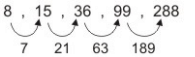8 + 7 x 1 = 15
15 + 7 x 3 = 36
36 + 7 x 32 = 99
36 + 7 x 33 = 288
So, 28 + 7 x 34 = 855

QUESTION: 49

A number is multiplied by 5 and 25 is added to it.The result is divided by 5 and the original number is subtracted from the same.The remainder will be

Solution:

Let the number be x Then,
Remainder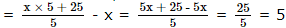QUESTION: 50
When 50% of one number is added to a second number,the second number increases to its four-thirds. What is the ratio between the first number and second number?
Solution:
QUESTION: 51
The 18th All India Whips’ Conference was organized in which city?
Solution:
QUESTION: 52
Two pipes can fill a tank in 2 hrs and 3 hrs separately, How many minutes they will take to fill the tank when both are opened together?
Solution:
QUESTION: 53
A man purchased a box full of pencils at the rate of 7 for Rs 9 and sold all of them at the rate of 8 for Rs 11. In this transaction, he gained Rs 10. How many pencils did the box contain?
Solution:
QUESTION: 54
If 0.75:x::5:8, then x is equal to:
Solution:
QUESTION: 55

A sum of Rs. 1550 was lent partly at 5% and partly at 8% simple interest. The total interest received after 3 year is Rs.300. The ratio of money lent at 5% to that at 8%

Solution:

Let the sum lent at 5% be x and 8% be 1550 - x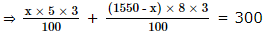⇒ 15x + 37200 - 24x = 30000
⇒ 7200 = 9x
⇒ x = 800
⇒ 1550 - x = 750
Ratio = 800 750 = 16 15
i.e. 16 : 15

QUESTION: 56
Zoospore is formed in
Solution:
QUESTION: 57

7500 + (1250 ÷ 50) = ?

Solution:
QUESTION: 58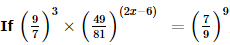, then the value of x is:

Solution:
QUESTION: 59
Name the team to win the 8th Hockey India Sub Junior (Women) National Championship 2018 (A Division)
Solution:
QUESTION: 60

The least number by which 2 x 6 x 7 x 7 should be multiplied so as to make it perfect square is

Solution:
QUESTION: 61
Below is given statement followed by two arguments numbered I and II. You have to consider the statement and the following arguments and decide which of the arguments is strong in the statement.
Statement :
Should there be recruitment in banks on the basis of past academic performance rather than through competitive examinations?
Arguments:
I. Yes, It will be beneficial for those candidates who are unable to bear the expenses of competitive examinations.
II. No, the past academic performance cannot be made the basis of recruitment because there is no uniformity in the assessment by the universities.
Solution:
QUESTION: 62
The average of values 0, 2, 4, 6, 8, ...., 2n is
Solution:
QUESTION: 63
In each question a statement followed by two assumptions numbered I and II is given. Consider the statement and decide which of the given assumption is implicit.
Statement : Most people who stop smoking gain weight.
Assumptions : I. If one stops smoking one will gain weight.
II. If one does not stop smoking one will not gain weight.
Solution:
QUESTION: 64
Below is given statement followed by three conclusions numbered I, II and III. You have to consider the statement and the following conclusions and decide which of the conclusions is follows in the statement :
Statements :a. All lions are tigers.
b. All tigers are leopards.
c. Some leopards are wolves.
d. No wolf is elephant.
Conclusions:I. No elephant is lion.
II. Some wolves are lions.
III. Some leopards are lions.
Solution:
QUESTION: 65
If 2x + y = 7 and x + 2y = 7 are the two regression lines respectively, then the correlation co-efficient between x and y is
Solution:
QUESTION: 66
Below are the statements followed by four conclusions numbered I,II,III and IV . You have to consider the statements and the following conclusions and decide which of the conclusion(s) follows the statement(s).
Statements:
a. All men are vertebrates.
b. Some Mammals are vertebrates.
Conclusions:
I. All men are mammals.
II. Some vertebrates are mammals.
III. All vertebrates are men
IV. All mammals are men.
Solution:
QUESTION: 67

The distance between two cities A and B is 330 km. A train starts from A at 8 a.m. and travels towards B at 60 km/hr. Another train starts from B at 9 a.m. and travels towards A at 75 km/hr. At what time do they meet?

Solution:

The two trains meet x hrs after 8 a.m.
Distance covered by 1st train in x hrs + Distance covered by 2nd train in (x - 1) hrs = 330 km
60x + 75 (x - 1) = 330
60x + 75x - 75 = 330
x = 405/135 = 3
So, they will meet 3 hrs past 8 a.m. i.e., 11 a.m.

QUESTION: 68
A completes a work in 12 days and B completes the same work in 24 days. If both of them work together, then the number of days required to complete the work will be
Solution:
QUESTION: 69
Arrange the following words in a meaningful sequences :
1. Organs
2. Cells
3. Organ system
4. Organism
5. Tissues
Solution:
QUESTION: 70
What is the volume of a cube (in cubic cm) whose diagonal measures 4√3 cm?
Solution:
QUESTION: 71
The Doppler's Effect has been made use of in determining
Solution:
QUESTION: 72
Choose one word which cannot be made from the letters of the given word
RECOMMENDATION
Solution:
QUESTION: 73
'With the elastic limit, extension is directly proportional to the tension', this is known as
Solution:
QUESTION: 74

Seven basketball matches were played between the 2 finalists, during National Basketball Competition. After the match, 9 players (spectators inclusive) were being interviewed. One or more players were interviewed from the teams viz. Andhra Pradesh, Tamil Nadu, Gujrat, Madhya Pradesh, Uttar Pradesh, Maharashtra, West Bengal, Bihar, Bihar and Orissa. The name of the team is also denotes the name of the state team. Additional information about the interviewee is as follow.
→ Each player has unique jersey number.
→ David Disouza with jersey number 31 plays for Andhra Pradesh and is also known as a 3 point shooter.
→ Rajeev Sinha with jersey number 23 is the eldest player and Subhash Divyundu is the heaviest player in National Basketball Competition.
→ Sachdev Sharma has jersey number 30, while Jayesh Patel has 40.
→ Rahul Jha is one of he dunkers and he is from ‘West Begal’.
→ The player from Uttar Pradesh and a Tamil Nadu have a single digit jersey number.
→ Both the players with jersey number 40 and 34 play for Gujrat.
→ Rahul Jha was interviewed after Ramesh Naydu, but wasn’t the last person to be interviewed.
→ Both the players from Tamil Nadu and the players from Gujrat and Orissa were interviewed one after the other, and so were the players from Uttar Pradesh, Maharashtra and West Bengal, and in that order.
→ Jersey (23) plays for Bihar.
→ The heaviest player with jersey number 34 plays for Orissa.
→ Ramesh Naydu is a ‘passer’ for Andhra Pradesh.
→ Jersey number 2 and 3 were the only single digit jerseys.

Q. Who wears jersey number 40?

Solution:
QUESTION: 75

Seven basketball matches were played between the 2 finalists, during National Basketball Competition. After the match, 9 players (spectators inclusive) were being interviewed. One or more players were interviewed from the teams viz. Andhra Pradesh, Tamil Nadu, Gujrat, Madhya Pradesh, Uttar Pradesh, Maharashtra, West Bengal, Bihar, Bihar and Orissa. The name of the team is also denotes the name of the state team. Additional information about the interviewee is as follow.
→ Each player has unique jersey number.
→ David Disouza with jersey number 31 plays for Andhra Pradesh and is also known as a 3 point shooter.
→ Rajeev Sinha with jersey number 23 is the eldest player and Subhash Divyundu is the heaviest player in National Basketball Competition.
→ Sachdev Sharma has jersey number 30, while Jayesh Patel has 40.
→ Rahul Jha is one of he dunkers and he is from ‘West Begal’.
→ The player from Uttar Pradesh and a Tamil Nadu have a single digit jersey number.
→ Both the players with jersey number 40 and 34 play for Gujrat.
→ Rahul Jha was interviewed after Ramesh Naydu, but wasn’t the last person to be interviewed.
→ Both the players from Tamil Nadu and the players from Gujrat and Orissa were interviewed one after the other, and so were the players from Uttar Pradesh, Maharashtra and West Bengal, and in that order.
→ Jersey (23) plays for Bihar.
→ The heaviest player with jersey number 34 plays for Orissa.
→ Ramesh Naydu is a ‘passer’ for Andhra Pradesh.Use Code STAYHOME200 and get INR 200 additional OFF Use Coupon Code